• python使用matplotlib可视化、自定义设置坐标轴的范围、自定义设置主坐标轴刻度和次坐标轴刻度（ticks）、...
多人点赞
2022-02-20 18:43:54

# python使用matplotlib可视化、自定义设置坐标轴的范围、自定义设置主坐标轴刻度和次坐标轴刻度（ticks）、自定义坐标轴刻度的显示样式、自定义坐标轴刻度数值的颜色以及小数点位数

目录python 数据挖掘 机器学习 人工智能
更多相关内容
• 此次我将通过一些示例演示如何将坐标轴刻度调整为你需要的位置与格式。 在介绍示例之前，我们最好先对 Matplotlib 图形的对象层级有更深入的理解。Matplotlib 的目标是用 Python 对象表现任意图形元素。例如，想想...
• 坐标轴刻度取值算法完整代码，生成比较优雅和人性化的刻度。
• 今天小编就为大家分享一篇python matplotlib绘图,修改坐标轴刻度为文字的实例，具有很好的参考价值，希望对大家有所帮助。一起跟随小编过来看看吧
• 修改python plot折线图的坐标轴刻度，这里修改为整数： 代码如下： from matplotlib import pyplot as plt import matplotlib.ticker as ticker import numpy as np def std_plot(): overall_std = [34.369, 21....
• 前言 matplotlib.pyplot是一些命令行风格函数的集合，使matplotlib以类似于MATLAB的方式工作。每个pyplot函数对一幅图片(figure)做一些改动：比如创建新图片，在图片创建一个新的作图区域(plotting area)，在一个...
• 主要介绍了Python设置matplotlib.plot的坐标轴刻度间隔以及刻度范围，具有一定的参考价值，感兴趣的小伙伴们可以参考一下
• 文章目录一、未设置横纵坐标轴刻度（默认情况下）二、修改坐标轴刻度 一、未设置横纵坐标轴刻度（默认情况下） 示例代码： # 修改x轴与y轴的刻度 import matplotlib.pyplot as plt lst1 = list(range(0,15)) lst2 = ...

# 一、未设置横纵坐标轴刻度（默认情况下）

示例代码1：

# 修改x轴与y轴的刻度
import matplotlib.pyplot as plt
lst1 = list(range(0,15))
lst2 = list(range(15,30))
plt.plot(lst1,lst2)
plt.show()


结果如下：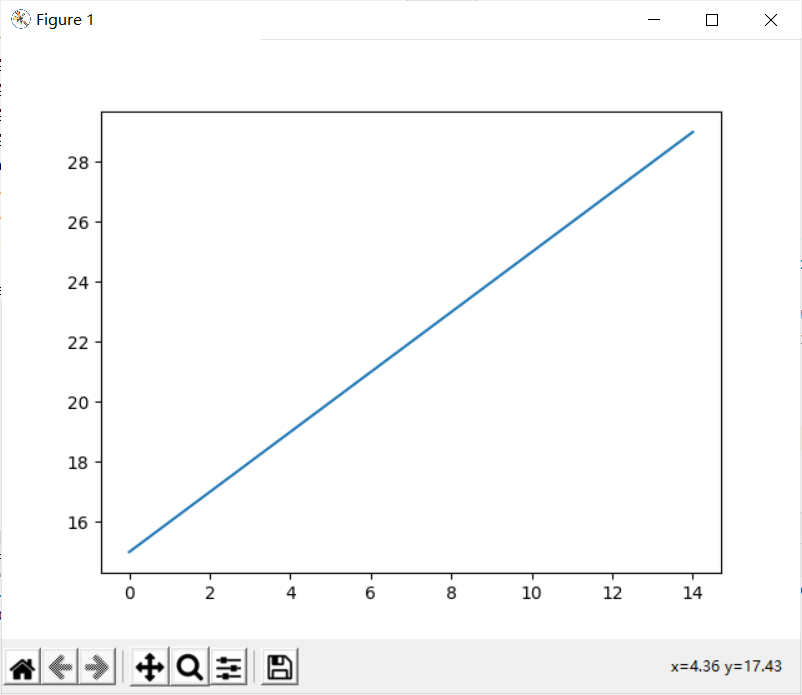此时上图中的坐标轴刻度间距为2

# 二、修改坐标轴刻度

通过添加以下代码对坐标轴刻度进行修改

from pylab import xticks,yticks,np
# 修改横坐标的刻度
xticks(np.linspace(0,15,16,endpoint=True))
# 修改纵坐标的刻度
yticks(np.linspace(15,30,16,endpoint=True))

linspace()中四个参数的意义(本人理解，可能有误！)：
第一个参数为坐标的起始位置
第二个参数为坐标的终止位置
第三个参数为将坐标分成多少份（该例中将0-15分成了16份，每刻度为1）
第四个参数为是否取最后一个点（默认是endpoint=False左开右闭）
"""


示例代码2：

# 修改x轴与y轴的刻度
import matplotlib.pyplot as plt
from pylab import xticks,yticks,np
lst1 = list(range(0,15))
lst2 = list(range(15,30))
"""
linspace()中四个参数的意义：
第一个参数为坐标的起始位置
第二个参数为坐标的终止位置
第三个参数为将坐标分成多少份（该例中将0-15分成了16份，每刻度为1）
第四个参数为是否取最后一个点（默认是endpoint=False左开右闭）
"""
# 修改横坐标的刻度
xticks(np.linspace(0,15,16,endpoint=True))
# 修改纵坐标的刻度
yticks(np.linspace(15,30,16,endpoint=True))
plt.plot(lst1,lst2)
plt.show()


结果如下：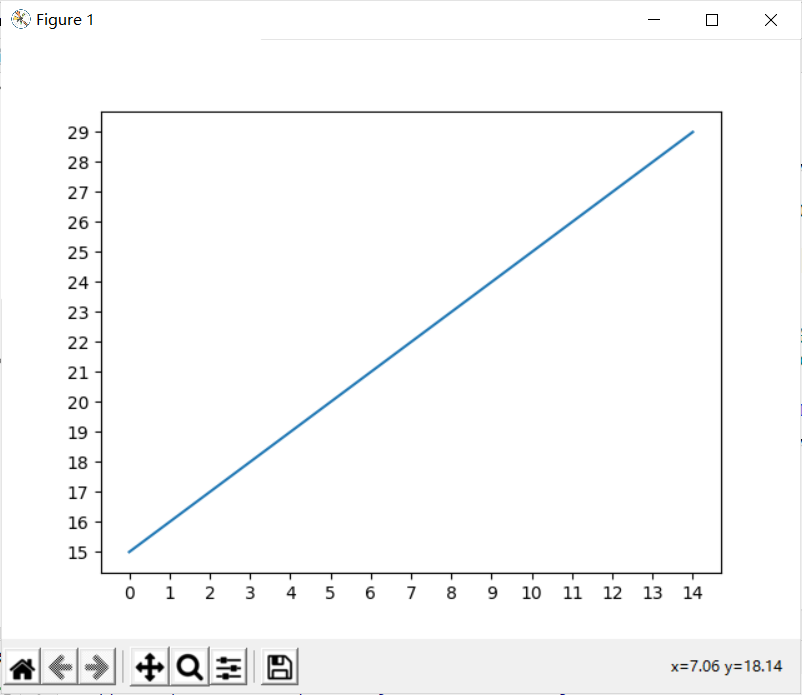此时上图中的坐标轴刻度间距为1

# 三、给对应的横坐标刻度加上标签文本

默认情况下刻度显示的文本就是刻度本身
如果要修改刻度的话，需要在plt.xticks()方法中在再添加一个参数,在示例代码2中添加如下代码

xticks_labels = ['11点{}分'.format(i) for i in range(0,16)]
# 修改横坐标的刻度,并且为横坐标上的每个刻度添加标签
xticks(np.linspace(0,15,16,endpoint=True),xticks_labels)


注意：plt.xticks()和plt.yticks()方法,在传递进去的第一个参数必须是数字,不能是字符串,如果是字符串吗,需要进行替换操作
示例代码3：

# 修改x轴与y轴的刻度
import matplotlib.pyplot as plt
from pylab import xticks,yticks,np
lst1 = list(range(0,15))
lst2 = list(range(15,30))
"""
linspace()中四个参数的意义：
第一个参数为坐标的起始位置
第二个参数为坐标的终止位置
第三个参数为将坐标分成多少份（该例中将0-15分成了16份，每刻度为1）
第四个参数为是否取最后一个点（默认是endpoint=False左开右闭）
"""
xticks_labels = ['11点{}分'.format(i) for i in range(0,16)]
# 修改横坐标的刻度
xticks(np.linspace(0,15,16,endpoint=True),xticks_labels)
# 修改纵坐标的刻度
yticks(np.linspace(15,30,16,endpoint=True))
plt.plot(lst1,lst2)
plt.show()


代码运行结果如下：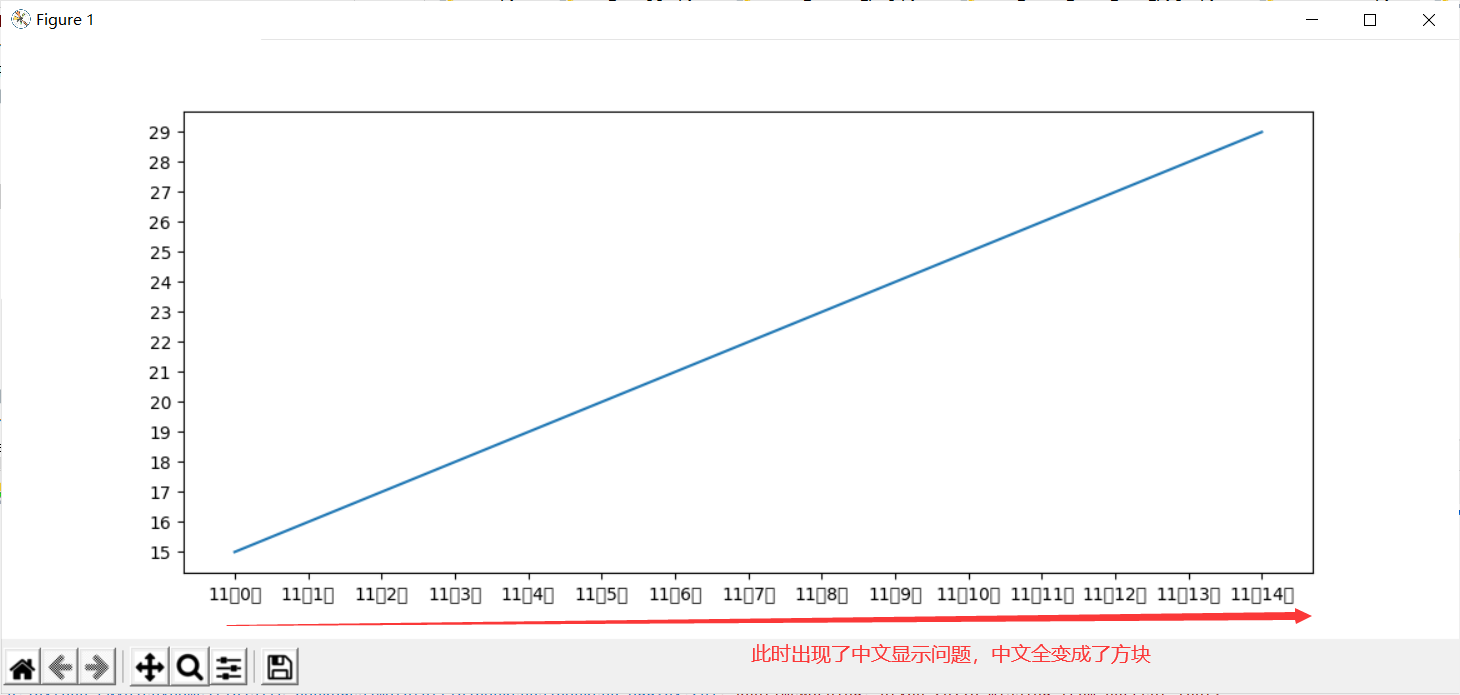在示例代码3中添加以下代码解决中文显示问题：

from pylab import *
mpl.rcParams['font.sans-serif'] = ['SimHei'] # 指定默认字体（解决中文无法显示的问题）
mpl.rcParams['axes.unicode_minus'] = False # 解决保存图像时负号“-”显示方块的问题


完整代码如下

# 修改x轴与y轴的刻度
import matplotlib.pyplot as plt
from pylab import xticks,yticks,np
from pylab import *
mpl.rcParams['font.sans-serif'] = ['SimHei'] # 指定默认字体（解决中文无法显示的问题）
mpl.rcParams['axes.unicode_minus'] = False # 解决保存图像时负号“-”显示方块的问题
lst1 = list(range(0,15))
lst2 = list(range(15,30))
"""
linspace()中四个参数的意义：
第一个参数为坐标的起始位置
第二个参数为坐标的终止位置
第三个参数为将坐标分成多少份（该例中将0-15分成了16份，每刻度为1）
第四个参数为是否取最后一个点（默认是endpoint=False左开右闭）
"""
xticks_labels = ['11点{}分'.format(i) for i in range(0,16)]
# 修改横坐标的刻度
xticks(np.linspace(0,15,16,endpoint=True),xticks_labels)
# 修改纵坐标的刻度
yticks(np.linspace(15,30,16,endpoint=True))
plt.plot(lst1,lst2)
plt.show()


代码运行结果如下：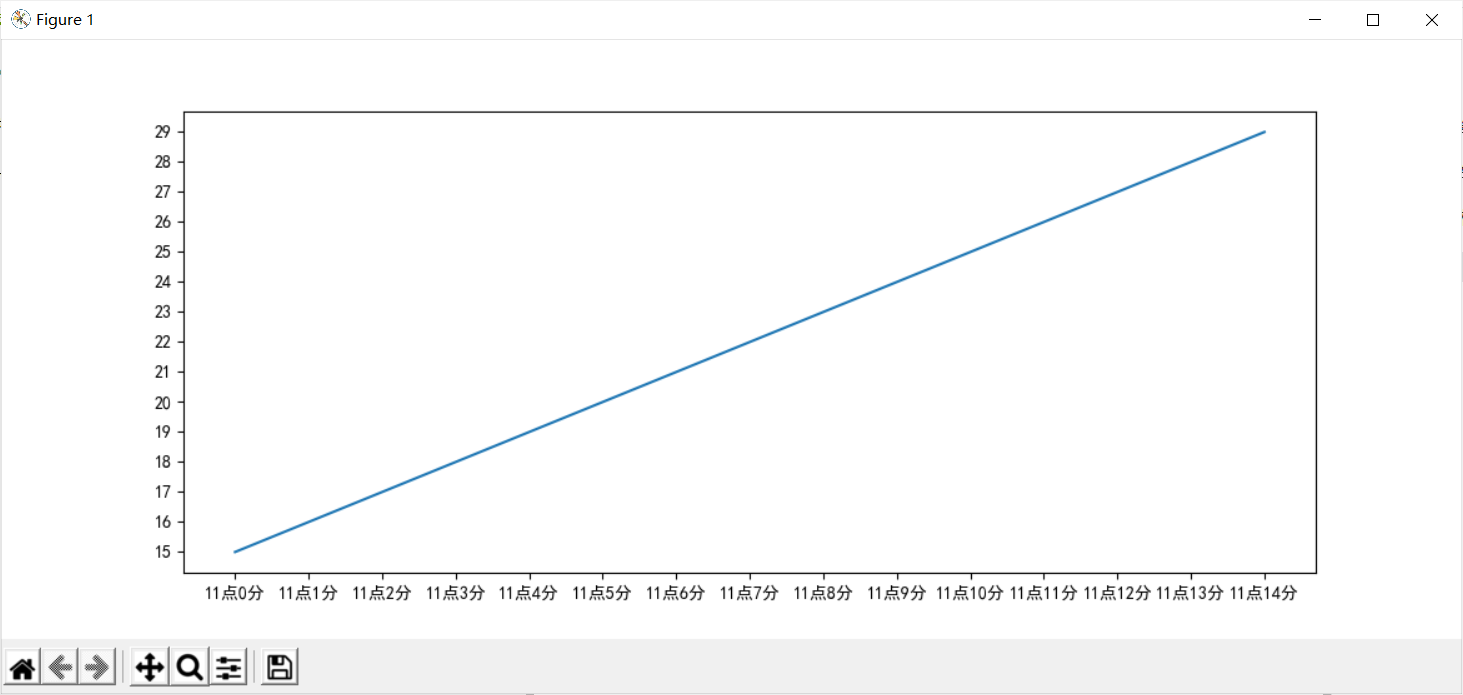展开全文python 开发语言 后端
• 【转载】Matlab 绘图 坐标轴 刻度原文出处...用matlab绘图函数plot,plot3等绘制的图形，其坐标轴的刻度可以通过手动方式修改，请问有没有什么函数可以实现坐标轴刻度的修改？str='E:cjf'x=[0:0.1:1];xlab=[1:11];fo...

【转载】Matlab 绘图 坐标轴 刻度

原文出处：http://blog.sina.com.cn/s/blog_4b1fb7610100q9ov.html

matlab中坐标轴的刻度如何设置？

用matlab绘图函数plot,plot3等绘制的图形，其坐标轴的刻度可以通过手动方式修改，请问有没有什么函数可以实现坐标轴刻度的修改？

str='E:cjf'

x=[0:0.1:1];

xlab=[1:11];

for i=1:10

plot([0 1],[0 1]);hold on;plot([0 1],[1 2]);

set(gca,'xtick',x);

set(gca,'xticklabel',xlab);

hold off;

saveas(gcf,[str num2str(i) '.bmp']);

end

补充一点，刻度不限制于数字。比如：Day = {'Sun','Mon','Tue','Wed','Thu','Fri','Sat'}；plot(1:7,1:7);

set(gca,'xticklabel',Day);EXAMPLE: Matlab中Tick(坐标轴刻度)的不同风格 http://crust.cn/?p=287

x=1:8;

subplot(2,2,1)

plot(x)

%tick style 0(auto)

subplot(2,2,2)

plot(x)

set(gca,'xtick',[1 3 6 8]);%style 1

set(gca,'ytick',[]);%style 2

subplot(2,2,3)

plot(x)

set(gca,'xtick',[1 3 6 8]);

set(gca,'xticklabel',sprintf('.4f|',get(gca,'xtick')));%style 3

set(gca,'ytick',[2 4 5 7]);

set(gca,'yticklabel',{'Two','Four','Five','Seven'});%style 4

subplot(2,2,4)

plot(x)

set(gca,'xminortick','on');%style 5

set(gca,'ticklength',[0.05 0.025]);%style 6

set(gca,'tickdir','out');%style 7

x=[1 1.53 4];

y=[1 2 3];

plot(x,y)

set(gca,'XTick',x)

set(gca,'XTickLabel',sprintf('%3.4f|',x))

set(gca,'YTick',y)

set(gca,'YTickLabel',sprintf('%+1.2f|',y))set(gca,'xtick'，[x起始坐标:间隔:x终点坐标]);   %设置x轴

set(gca,'ytick'，[y起始坐标:间隔:y终点坐标]);   %设置y轴

matlab非等长坐标

set(gca,'xtick',[0:10:100,140:40:400])关于label

大家好，有个问题想向各位请教一下，在此先行谢过。

是这样的，当我在采用plot3等三维作图函数时，调用xlabel，ylabel，zlabel等作用后的结果是x轴和y轴的文字沿着水平方向，如果文字的方向能够设置得跟轴的方向一致的话就美观多了。

我目前采用的方法是:

close all;

figure;

plot3(10, 20, 30, 'Marker', 'o', 'MarkerSize', 20);

xlabel('中华人民共和国');

set(get(gca, 'XLabel'), 'Rotation', 15);

set(get(gca, 'XLabel'), 'Color', 'r');

ylabel('中华人民共和国');

set(get(gca, 'YLabel'), 'Rotation', -23);

set(get(gca, 'YLabel'), 'Color', 'g');

zlabel('中华人民共和国');

set(get(gca, 'ZLabel'), 'Color', 'b');

其中旋转的角度15和-23是我凭感觉试出来的，感觉很笨拙，不知道有没有什么更好的办法，能够一步准确的设置到位？希望能够得到热心朋友的帮助，万分感谢。关于竖向或倾斜文本

plot(0:pi/20:2*pi,sin(0:pi/20:2*pi))

text(pi,0,' leftarrow sin(pi)','FontSize',18,'rotation',90)刚在一个matlab牛人的博客上看到一个axes函数中用'position'参数问题，

大概是在一个图中，不同位置画一些图形

EXAMPLE：

x = 0 : 0.01 : 2 * pi;

y1 = sin(x); y2 = cos(x); y3 = sin(x) + cos(x);

plot(x, y1)

axes('position', [0.55 0.65 0.3 0.2])

plot(x, y2)

xlabel('y2 = cos(x)');

axes('position', [0.15 0.15 0.3 0.2])

plot(x, y3)

xlabel(' y3 = sin(x) + cos(x)');set(gca,'Position',[left bottom width height]

set(gca,'Position',[0.15 0.15 0.75 0.75])       %设置图像的位置position设置文字的位置

EXAMPLE：

x=0:0.01:2*pi;

y=sin(x);

plot(x,y);

xlabel('XLABEL','position',[2 -1.15 1]);

ylabel('YLABEL','position',[-0.5 0 1]);

title('TITLE:Y=SIN(X)','position',[5 0.6 1],'rotation',45);可以scrsz=get(0,'ScreenSize')检查LZ荧幕的pixel数h0=figure('toolbar','none',    'position',[198 56 350 300],    'name','实例01');

这个position具体的范围是多少

198 56 350 300这几个数字是什么意思

h1=axes('parent',h0,   'visible','off');

这句有什么作用

请各位朋友帮忙指点一下！！答：

[物体(此为figure)距离参照物(此为屏幕)左下角的x轴距离，y轴距离，物体的宽度，物体的高度]

h1=axes('parent',h0,   'visible','off');

应该是在h0中创建一个axes对象，且设置其visible属性为off自定义设置坐标轴内容

请教各位大虾：

matlab 画出柱状图以后，想用字符串表示横坐标，对应那些柱，怎么做？

比如1,2,3,4,5想改成B,A,BA,BCD,CD

自动生成的全是数字，改了半天也没改好，郁闷

找到命令set(gca,'xtick',[ ])可以把x轴刻度清空,但怎么在原来柱状图显示1 2 3 4 5 的地方显示上 AB CD EF GH IJ 呢????答：set(gca,'xtick',['AB','CD']);

EXAMPLE:

x=20:10:20000;

y=rand(size(x));

semilogx(x,y);

set(gca,'XLim',[20 20000]);

set(gca,'XMinorTick','off');

set(gca,'XTick',[20 31.5 63 125 250 500 1000 2000 4000 8000 16000]);

set(gca,'XGrid','on');

set(gca,'XMinorGrid','off');ezplot('x^3+x^2+x+5',[1,100])

set(gca,'xtick',1:100,'xticklabel',mod(1:10,10)) %xtick就是你要在哪些地方显示刻度，xticklabel就是显示什么文字格式设置

figure_FontSize=12;

set(get(gca,'XLabel'),'FontSize',figure_FontSize,'Vertical','top');

set(get(gca,'YLabel'),'FontSize',figure_FontSize,'Vertical','middle');

set(findobj('FontSize',12),'FontSize',figure_FontSize);

%这4句是将字体大小改为8号字，在小图里很清晰

%set(gcf,'Position',[100 100 260 220]);

%这句是设置绘图的大小，不需要到word里再调整大小。我给的参数，图的大小是7cm

%set(gca,'Position',[.13 .17 .80 .74]);

%这句是设置xy轴在图片中占的比例，可能需要自己微调。

%set(findobj(get(gca,'Children'),'LineWidth',0.5),'LineWidth',2);

%这句是将线宽改为2

set(gca, 'Fontname', 'Times newman', 'Fontsize', 12);

%设置图片的字体类型和字号大小的。展开全文• 今天小编给大家讲解excel2013调节图表坐标轴刻度的相关教程，有需要或者有兴趣的朋友们可以看一看下文，相信对大家会有所帮助的。excel2013调节图表坐标轴刻度的相关教程启动excel2013，打开表格，看到一整列数据，...

今天小编给大家讲解excel2013调节图表坐标轴刻度的相关教程，有需要或者有兴趣的朋友们可以看一看下文，相信对大家会有所帮助的。

excel2013调节图表坐标轴刻度的相关教程启动excel2013，打开表格，看到一整列数据，将其改为横排样式，单击菜单栏--插入--数据透视图--数据透视图。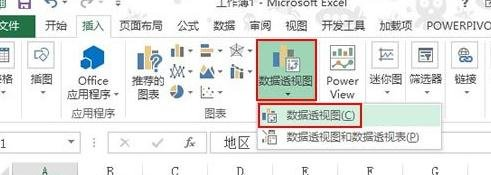选择表格区域，下面勾选新建工作表，这样不会影响原来表格的版式布局。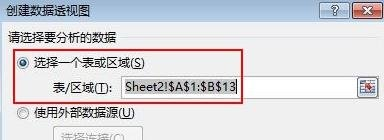确定后，弹出数据透视图字段，勾选地区和数据，并拖动数据到图例里。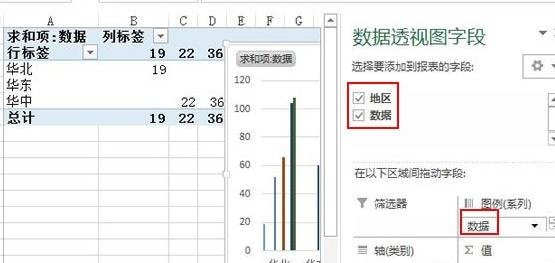选中左侧表格里的数据区域，华北、华东和华中，复制。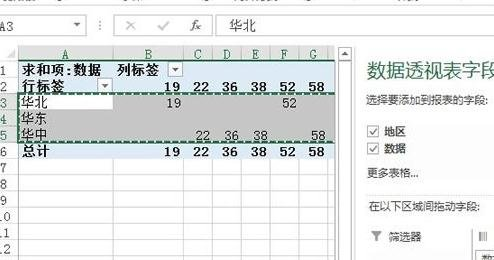返回到原表格，粘贴到任意一个位置，如图所示。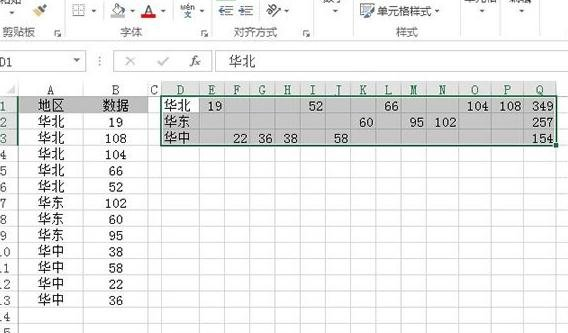删除Q列，也就是总计的那列，选中D1：P3区域，单击F5键，弹出定位对话框，点击定位条件按钮。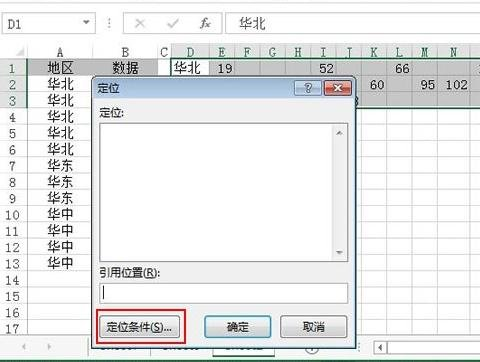在定位条件界面中，勾选空值，确定。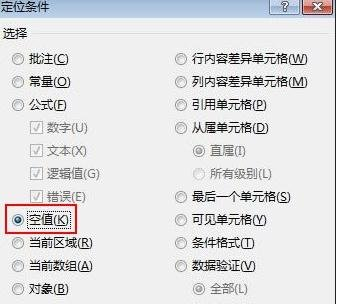点击菜单栏--开始--单元格--删除--删除单元格。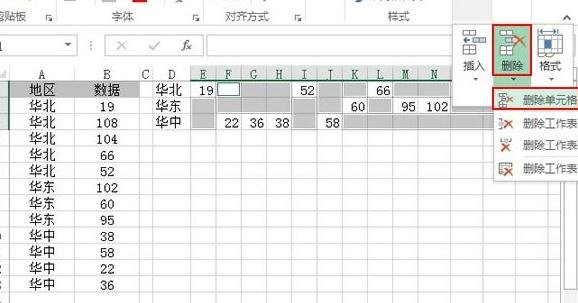勾选右侧单元格左移，确定。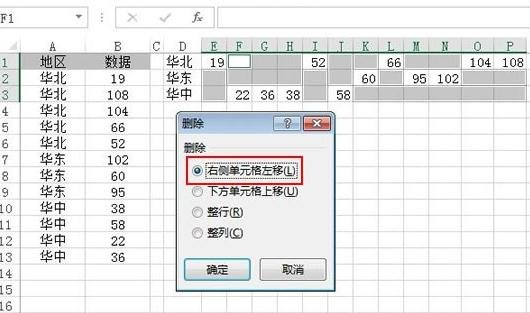最终效果如下所示：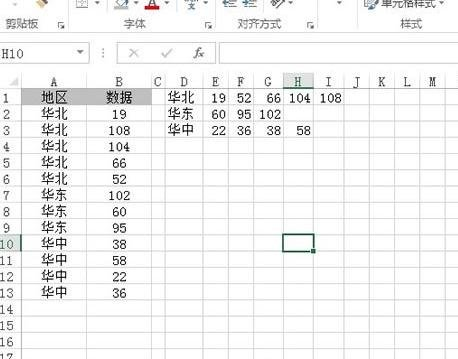excel相关攻略推荐:

以上这里为各位分享了excel2013调节图表坐标轴刻度的操作内容。有需要的朋友赶快来看看本篇文章吧。

展开全文• a=linspace(1,2,10)plot(a,'--pr','linewidth',1.5,'MarkerEdgeColor','r','MarkerFaceColor','m','MarkerSize',10)legend('a','Location','best')title('a','FontName','Times NewRoman','FontWeight','Bold','Font...
• matlab坐标轴操作大全_电脑基础知识_IT/计算机_专业资料。 坐标轴标注: 1. 控制坐标轴尺度长度:set(gca,'XLim',[-pi/2 pi]) 2. 定制自己想标注的刻度: ......axis normal 自动调整纵横轴比例...坐标轴刻度设置 set.....
• python使用matplotlib可视化、自定义设置坐标轴的范围、自定义设置主坐标轴刻度和次坐标轴刻度、自定义坐标轴刻度的显示样式、自定义坐标轴刻度数值的颜色以及小数点位数、添加坐标轴刻度网格线、自定义移动坐标轴的...python 数据挖掘 机器学习 人工智能
• ## ggplot2修改坐标轴刻度

万次阅读 多人点赞 2021-01-14 12:52:50
如何修改坐标轴刻度标签（大小、字体、颜色、加粗、位置、角度） 如何修改坐标轴刻度间隔 如何去掉网格线 如何去掉刻度标签 如何去掉刻度线 如何去掉外层边框 如何再加上X轴、Y轴（无刻度、无标签） （更...
• 总结matplotlib绘图如何设置坐标轴刻度大小和刻度。 上代码： from pylab import * from matplotlib.ticker import MultipleLocator, FormatStrFormatter xmajorLocator = MultipleLocator(20) #将x主刻度标签设置...
• 可以看到坐标轴刻度其实是错误的。 3、如图所示，添加以下代码： set(gca,'XTick',0:pi/2:2*pi); set(gca,'XTicklabel',{'0','π/2','π','3π/2','2π'}) 设置对应的x坐标轴刻度 4、如图所示，运行图形...计算机视觉 深度学习 机器学习
• 一、坐标轴的标注(1)均匀标注均匀标注需要确定间隔的大小x=0:2*pi/100:2*pi;y=sin(x);xlim([0:2*pi];set(gca,'Xtick',[0:0.5:2*pi]) %间隔为0.5(2)不均匀标注比如x轴的数值范围为：0-10，而主要标注在x=2,x=5,x=7,x=...
• 怎么让excel表格坐标轴表上刻度解决案：1、图拖鼠标选要建立图标数据2、菜单栏：插入--图选择种式3、插入图表先点击纵坐标轴选看坐标轴四周现四圆叫做控制点4、右键单击坐标轴选择【设置坐标轴格式】5、打坐标轴格式...
• R语言ggplot2可视化增加坐标轴的刻度数实战：自定义坐标轴刻度粒度、增加坐标轴刻度的粒度、更加精细地表达 目录 R语言ggplot2可视化增加坐标轴的刻度数实战：自定义坐标轴刻度粒度、增加坐标轴刻度的粒度、更加...
• matplotlib，利用pyplot与面向对象的api两种方式设置坐标轴刻度的个数以及标签matplotlib
• 指定坐标轴刻度值和标签 更改刻度值位置和标签 旋转刻度标签 更改刻度标签格式 用于分别控制各个坐标轴的标尺对象 使用标尺对象控制指数标签中的值 指定坐标轴刻度值和标签 自定义沿坐标轴的刻度值和标签有助...matlab 开发语言
• matplotlib中实现坐标刻度应用的函数是： matplotlib.pyplot.xticks和matplotlib.pyplot.yticks xticks()中有3个参数： xticks(locs, [labels], **kwargs) # Set locations and labels locs参数为数组参数(array_...
• 一、先画坐标轴，然后写上数字或刻度短线，不用管格式位置 二、选中数字，形状格式->对齐->底部对齐 效果如下 三、选中数字，形状格式->对齐->横向分散 效果 ......
• 此次我将通过一些示例演示如何将坐标轴刻度调整为你需要的位置与格式。 在介绍示例之前，我们最好先对 Matplotlib 图形的对象层级有更深入的理解。Matplotlib 的目标是用 Python 对象表现任意图形元素。例如，想想...python
• import matplotlib.pyplot as plt plt.xticks([1, 2, 3, 4, 5, 6, 7, 8]) plt.tick_params(labelsize=13) #刻度字体大小13matplotlib...